﻿ St. Mary's H.S. Physics - Intro to Friction Notes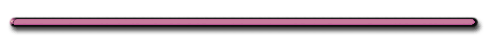HomeSt. Mary's H.S. Physics YouTube Channel

Kinetic Friction or Static?

Name two characteristics of this friction.

FRICTION force vector
always drawn opposite to motion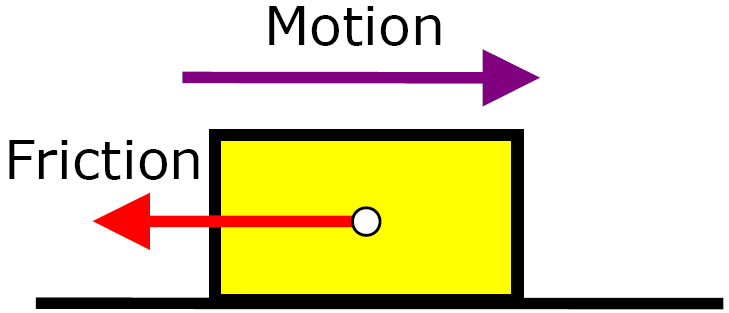This object below is moving to the right.  Describe its velocity.Object's velocity is increasing.This object is moving. Describe its velocity. Which way is it moving?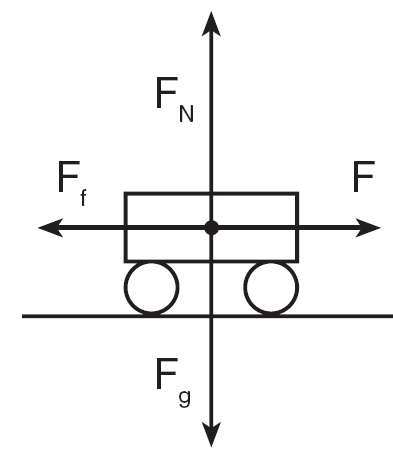Constant Velocity, Fnet = 0

The car is moving to the right.

*** At Constant Velocity:

Fapplied = Friction Force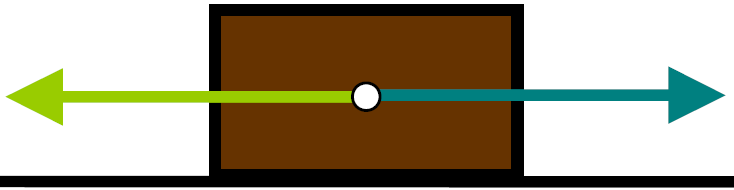a = 0

Fnet = 0

*** When object is moving
on a horizontal surface,
the normal force equals .....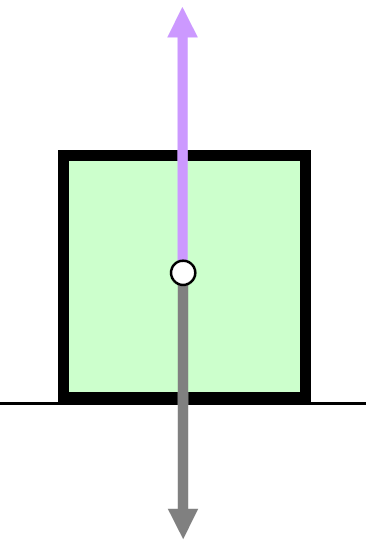... the weight force.

Fn = weight force

= w = mg

On a horizontal surface:

fs= Fnus = FgUs = mgUs

fk= FnUk = FgUk = mgUkBoth blocks are being pulled at constant velocity. What is the reading on the force scale on the right?Hint: fk = mgUkf = 10 N, m, g, Uk don't change when blocks are stacked!Ex 1) If an object is moving on a horizontal surface and you doubled the weight force on the object, how would that change the kinetic friction force?

(Hint: weight force = normal force on horizontal surface.)

kinetic Friction
Would Double

On a horizontal surface

Static Friction equation

fs= Fnus = Fgus = mgus

Kinetic friction equation

fk= FnUk = FgUk = mgUk

1) What would happen to the kinetic friction (fk) if:

a) Uk (the roughness number) were halved?

fk= FnUk

f would be halved

b) tripled the weight force (F)?

fk= wUk

f would be tripled

c) turned the block on its' side?

fk= FnUk = wUk = mgUk

f would be unchangedFriction Questions